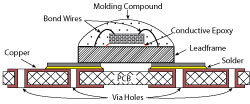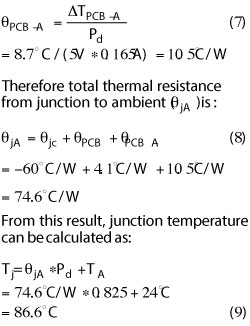Figure 1 Cross section of a MMIC component.

Temperature has a direct impact on the operating performance and reliability of semiconductor devices. Devices that operate at high junction temperatures for long periods of time can sustain permanent damage sooner, compromising reliability and shortening operating lifetime. For thermally related failures, the life of a MMIC component is directly related to the temperature at the hot spot of the semiconductor die in the device which is mounted on a printed circuit board (PCB). It is therefore critical that designers understand the thermal properties of a PCB design for a given MMIC device in order to minimize thermal resistance and temperature rise.

This article discusses a simple method for computing thermal resistance between a MMIC amplifier and the ambient environment. The temperature rise from the PCB to case is calculated based on the thermal resistance of the PCB layout and compared with measured temperatures using thermal photography to validate the accuracy of the calculation method.

CALCULATION

A packaged MMIC component consists of a semiconductor die mounted on a lead frame as shown in Figure 1. The die is attached to the lead frame using conductive epoxy and electrical connections from the die to the lead frame are made using wire bonding. The die is covered with a plastic molding compound to protect it from environmental damage and contamination.

Thermal resistance is defined as the ratio of temperature rise to the power dissipation and is expressed in °C/W. The thermal resistance from the hot spot on the die to external ambient consists of a series of thermal resistances:

• Hotspot on the die to bottom of die (θdie)
• Conductive epoxy (θce)
• Solder on PCB (θsl)
• Top of PCB to bottom surface (θPCB)
• PCB to ambient (θPCB-A)

The sum of thermal resistances of the die, conductive epoxy and lead frame is usually called θjc or thermal resistance from junction to case:This is typically provided by component manufacturers. An example is the Mini Circuits PHA-1+ amplifier for which θjc is 60°C/W.Figure 2 Suggested MMIC amplifier PCB layout.

The contribution of solder on the PCB (θsl) is small and not discussed in this article. What remains to be determined is the thermal resistance of the PCB which is dependent on PCB design. A thermal design using the PHA-1+ as an example is described.

The amplifier operates on a single +5V power supply and is housed in an industry standard SOT-89 package. The suggested PCB layout is shown in Figure 2. The layout must accommodate both the electrical requirements of the device (such as proper line impedance, DC bias, grounding, bypassing, etc.) and the thermal requirements. Via holes in the PCB play an important role in providing good electrical and thermal paths to ground. The recommended PCB layout consists of 7 via holes under and around the amplifier unit.

The thermal resistance (θ) of a single via hole can be calculated using Equation 2:Where:
l = Length of the via hole (m)
A = Area of via hole cross section (m2)
k= Thermal conductivity (W/m-K) = 385W/m-K (for copper)

For an unfilled via:Where:
do = Outer diameter of the via (m)
di = Inner diameter of the via (m)

Note that the difference between do and di is the copper thickness.Figure 3 MMIC amplifier test board.

Substituting Equation 3 into Equation 2, the thermal resistance of a single copper via is expressed as:Equation 4 implies that θdecreases as:

• Length of the via or PCB thickness decreases
• Via diameter (do) increases
• Copper thickness in the via (do – di) increases

Each of the 7 via holes in the suggested PCB layout of Figure 2 has a 0.02" outer diameter (do) with a copper thickness of 0.0014", resulting in an inner diameter (di) of 0.0172". PCB thickness is 0.020". Also, given a 0.0014" copper thickness on the top and bottom of the PCB, the length of the via (l) is 0.0228".

Substituting these parameters into Equation 4, the thermal resistance of a 20-mil diameter unfilled via hole is determined to be 29°C/W. For 7 via holes, we assume the thermal resistance is calculated as if they were in parallel to simplify the calculation; therefore, the thermal resistance for 7 vias in parallel is 4.1°C/W. This method does not take into account the spatial distribution of the vias, but it provides a good first order approximation.Figure 4 Thermal image to determine case, PCB, ground lug and MMIC body temperature.

Given that thermal resistance is the ratio of temperature rise to power dissipated, the value for thermal resistance allows us to predict the temperature rise as:Where Pd is power dissipation defined as the product of device voltage and current. For a PHA-1+ amplifier, the device voltage is +5V and current is 0.165A, so power dissipation is 0.825 W. The temperature rise is therefore:This is a first order approximation temperature rise from the device case to the ground lug.

MEASUREMENTS

To validate the accuracy of these calculations, a +5V supply is applied to the DC terminal of the test circuit shown in Figure 3, with measured DC current of 0.165A. After temperature stabilization, a thermal photograph is taken of the case, PCB, ground lug and amplifier body (see Figure 4). Table 1 compares measurement results with the calculations.

The measured (mean) temperature of the case (assuming it is the same as the bottom of the PCB) is 32.7°C; the measured (mean) temperature of the ground lug is 36.1°C. Therefore the measured temperature rise from case to ground lug is 3.4°C, which is the same as the computed value. The method for calculating thermal resistance shown in this article therefore allows designers to predict temperature rise without the need for sophisticated thermal analysis tools and software. This is a useful tool in designing a PCB to minimize thermal resistance.The calculation allows accurate prediction of thermal resistance from PCB to case. Often, thermal resistance from junction to ambient is needed. For the case of the PHA-1+, given an ambient temperature of 24°C and measured case temperature of 32.7°C, the difference between case and ambient temperature is ∆TPCB-A = 8.7°C.

The thermal resistance from case (or bottom of PCB) to ambient is:Conclusion

The procedure demonstrated in this article can be used to compute thermal resistance of the PCB and junction temperature in PCB design. In general, the thermal resistance of vias can be minimized by increasing number of vias, via outer diameter and/or copper thickness. It can also be decreased by decreasing via length or PCB thickness.